This method allows you add days, time, years, hours, seconds etc., on the moment object.

### Syntax

```moment().add(Number, String);
```

The following table shows the lists of keys/shorthand which you can use with add method.

Key Shorthand
years y
quarters Q
months M
weeks w
days d
hours h
minutes m
seconds s
milliseconds ms

You can make use of key or shorthand unit inside the add method as follows −

### Example

```moment.add(5, 'days'); or moment.add(5, 'd');
```

Let see a working example which shows to add days to the current date using add method.

### Example

```<html>
<style>
div { border: solid 1px #ccc;
font-family: "Segoe UI",Arial,sans-serif;
width: 75%;
}
</style>
<body>
<div style="font-size:25px" id="currentdate"></div>
<br/>
<br/>
<div style="font-size:25px" id="changeddate"></div>
<script type="text/JavaScript">
var day = moment(); //outputs current date.
document.getElementById("currentdate").innerHTML = "Current Date: " + day._d;
var changeddate = moment().add(5, 'days'); // adding 5 days to current date.
document.getElementById("changeddate").innerHTML = "Output after adding 5 days: " + changeddate._d;
</script>
</body>
</html>
```

### Output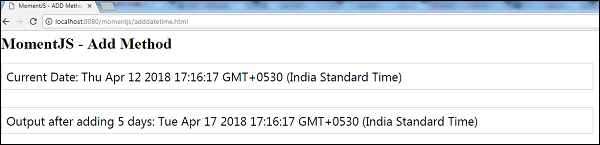Note that the above code displays the current date and the date after adding 5 days to it.

You can also use the key with add method as follows −

```var changeddate = moment().add(5, 'days'); // adding 5 days to current date.
```

### Example

Let see an example which adds 5 hours to the given date −

```var changeddate = moment([2017, 2, 31]).add(5, 'hours');
```

### Output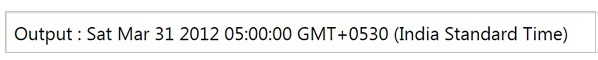If there are multiple additions to be done to the date, you can do it using add method chaining or using object literal.

Consider you want to add days and months to the current date. It can be done using method chaining as shown in below example −

### Example

```var changeddate = moment().add(5, 'days').add(2, 'months');.
```

### Output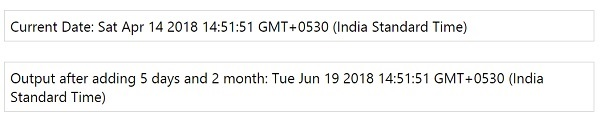To add days and months to the current date, we can use method chaining as follows −

```var changeddate = moment().add(5, 'days').add(2, 'months');.
```

We can also use key as shown in the code given below −

```var changeddate = moment().add(5, 'd').add(2, 'M');
```

Using this technique, you can use object literal for adding multiple keys to the current date.

### Example

```var changeddate = moment().add({ days: 5, months: 2 });
```

### Output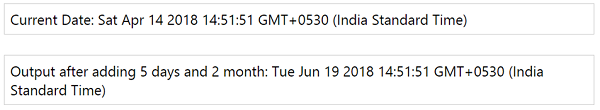The object method is used as follows −

```var changeddate = moment().add({ days: 5, months: 2 });
```

You can also use keys in the object form as follows −

```var changeddate = moment().add({ d: 5, M: 2 });
```

In case, we need to add days or months to a given date, the same can be done as shown below −

### Example

```var changeddate = moment([2014, 10, 10]).add({ d: 5, M: 2 });
```

### Output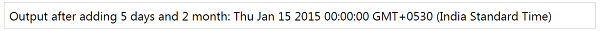We have added 5 days and 2 months to the date 10/10/2014 which gives the output as 15/01/2015.

We can also use duration method to add days, months, years, time etc. to a given date.

Observe the following example that shows how to add 5 weeks to a given date using duration −

### Example

```var duration = moment.duration({ 'weeks': 5 });
var changeddate = moment([2012, 0, 31]).add(duration);
```

Note that we have added 5 weeks to 31/01/2012 and thus the output is as follows −

### Output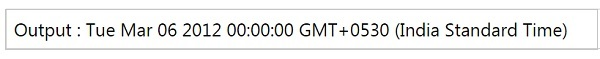### Special Cases for Months and Years

In case we are trying to add months to the date whose days are greater than the months added, it will take the last day of the month which is added to the date.

### Example

```var changeddate = moment([2017, 0, 31]).add(1, 'months');
```

In the above example, we are adding one month to 31/01/2017, now since February has 28 days it takes the last day of February and displays the date as shown below −

### Output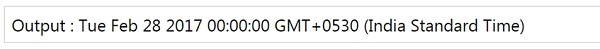momentjs_manipulate_date_and_time.htm